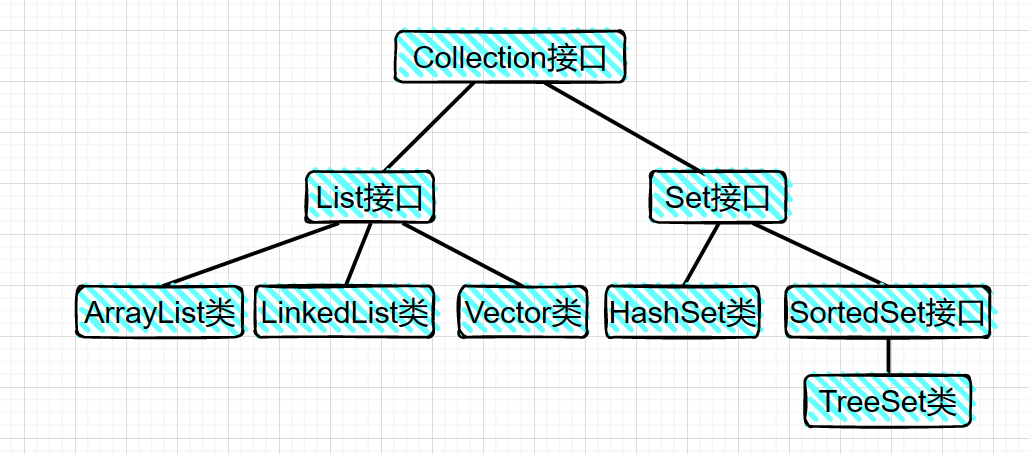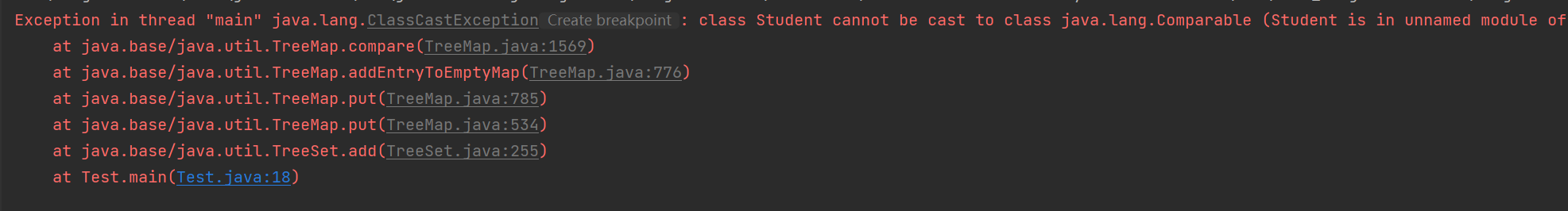### 文章目录

• 1. 概念
• 2. 集合和数组的区别
• 3. 集合的体系结构
• 4. Collection父接口
• 5. List 子接口
• 6. List 实现类
• 6.1 ArrayList 类
• 6.2 Vector 类
• 7. Set 子接口
• 8. Set 实现类
• 8.1 HashSet 类
• 8.2 TreeSet 类
• 9. Collections 工具类
• Java编程基础教程系列

# 1. 概念# 3. 集合的体系结构

Java中封装了很多的集合类，接下类的一段时间，我们就要一起探讨 Java 集合类的学习。由于Java集合类的知识繁杂，所以我们要先了解 Java 集合的体系结构，然后进行深入的学习。

Java集合结构庞大，其主要分为两大类，单列集合 Collection 和双列集合 map。所谓的单列集合是一次只能添加一个数据，而双列集合就是一次可以添加一对数据。# 4. Collection父接口

Collection 接口位于整个集合体系的最顶层，是一个根接口。 JDK 不提供此接口的任何直接实现，它提供了更具体的子接口的实现，如 Set 和 List ，两个接口具有不同的功能。

Lsit 接口的特点是添加的元素有序，可重复，有索引。有序指的是存取顺序，可重复是指集合中允许重复元素的存在，有索引是指每个元素都有对应的索引。 Set 接口添加的元素的特点相反，无序，不可重复，无索引。

Interface Collection 表示一组任意类型的对象。 一些集合允许重复元素，而其他集合不允许。我们主要从添加元素删除元素遍历集合等方面学习 Collection 的使用。

``boolean add(Object obj) //添加一个对象。boolean addAll(Collection c) //讲一个集合中的所有对象添加到此集合中。void clear() //清空此集合中的所有对象。boolean contains(Object o) //检查此集合中是否包含o对象。boolean equals(Object o) //比较此集合是否与指定对象相等。boolean isEmpty() //判断此集合是否为空。boolean remove(Object o) //在此集合中移除o对象。int size() //返回此集合中的元素个数。Object[] toArray() //姜此集合转换成数组。``

``import java.util.ArrayList;import java.util.Collection;import java.util.Iterator;public class Test {    public static void main(String[] args) { //创建集合 Collection collection=new ArrayList(); //添加元素 collection.add("张三"); collection.add("李四"); //打印元素个数 System.out.println("元素个数:"+collection.size()); //打印集合 System.out.println(collection); //删除元素 collection.remove("张三"); System.out.println("此时元素个数:"+collection.size()); //遍历集合元素 //1.增强for for(Object object :collection){     System.out.println(object); } //2.迭代器 Iterator iterator=collection.iterator(); while(iterator.hasNext()){     String s=(String)iterator.next();     System.out.println(s); }    }}``

# 5. List 子接口

List 接口继承自 Collection 接口，其具有有序，可重复，有索引的特点。我们可以使用索引来对集合元素进行操作。除了 Collection 接口中的方法外，List 还另外的定义了很多方法来对集合元素进行操作。

``import java.util.ArrayList;import java.util.List;import java.util.ListIterator;public class Test {    public static void main(String[] args) { //创建集合 List list = new ArrayList<>(); //添加元素 list.add("李四"); list.add("王五"); list.add("小张"); list.add("小明"); list.add("小王"); list.add(0, "张三"); //打印集合元素个数 System.out.println(list.size()); //打印集合元素 System.out.println(list); //删除集合元素 list.remove(2); System.out.println(list); //遍历集合元素 for (int i = 0; i < list.size(); i++) {     System.out.println(list.get(i)); } //使用列表迭代器遍历集合元素 ListIterator lit = list.listIterator(); while (lit.hasNext()) {     System.out.println(lit.nextIndex() + ":" + lit.next()); } while (lit.hasPrevious()) {     System.out.println(lit.previousIndex() + ":" + lit.previous()); } //判断 System.out.println(list.contains("张三")); System.out.println(list.isEmpty()); //获取元素位置 System.out.println(list.indexOf("李四")); //获取其子集，左闭右开 List list1 = list.subList(1, 3); System.out.println(list1);    }}``

# 6. List 实现类

## 6.1 ArrayList 类

ArrayList 实现类集合是使用数组实现的，其特点是查询快，增删慢。运行效率快，但是线程不安全。

ArrayList 实现了 List 接口，而 List 接口继承自 Collection 接口，所以它继承了两者的方法，。

``import java.util.ArrayList;import java.util.List;import java.util.ListIterator;public class Test {    public static void main(String[] args) { //创建集合 ArrayList arrayList=new ArrayList(); //添加元素 Student s1=new Student("张三",18); Student s2=new Student("李四",19); Student s3=new Student("王五",20); arrayList.add(s1); arrayList.add(s2); arrayList.add(s3); //打印集合 System.out.println(arrayList.size()); System.out.println(arrayList.toString()); //删除元素 arrayList.remove(s1); System.out.println(arrayList.size()); System.out.println(arrayList.toString()); //使用迭代器遍历 ListIterator lt=arrayList.listIterator(); while (lt.hasNext()){     Student s=(Student) lt.next();     System.out.println(s); } //判断 System.out.println(arrayList.isEmpty()); System.out.println(arrayList.contains(s1)); //查找 System.out.println(arrayList.indexOf(s2));    }}``

``/** 学生类*/public class Student {    private String name;    private int age;    public Student(String name, int age) { super(); this.name = name; this.age = age;    }    public String getName() { return name;    }    public void setName(String name) { this.name = name;    }    public int getAge() { return age;    }    public void setAge(int age) { this.age = age;    }    @Override    public String toString() { return "Student [name=" + name + ", age=" + age +"]";    }}``

## 6.2 Vector 类

Vector 实现类集合也是采用数组实现的，其不同于 ArrayList 的是运行效率较慢，但是线程安全。

Vector 实现了 List 接口，而 List 接口继承自 Collection 接口，所以它继承了两者的方法， 因为在开发中这个类已经不在常用了，所以只对类本身新增的几个方法讲解一下。

``import java.util.Vector;public class Test {    public static void main(String[] args) { //创建集合 Vector vector=new Vector(); //添加元素 vector.add("张三"); vector.add("李四"); vector.add("王五"); //增加的方法 System.out.println(vector.firstElement()); System.out.println(vector.lastElement()); System.out.println(vector.elementAt(2));    }}``# 7. Set 子接口

Set 接口位于 java.util 包中，Set 是一个无序集合，即存入和取出数据的顺序不一定相同。Set 集合中不可以添加重复元素。

Set 接口除了继承自 Collection 接口的所有方法如 add，equals 和 hashCode 方法外 ， 还增加了其他规定。 下面使用往集合中添加学生类举例：

``/** 学生类*/public class Student {    private String name;    private int age;    public Student(String name, int age) { super(); this.name = name; this.age = age;    }    public String getName() { return name;    }    public void setName(String name) { this.name = name;    }    public int getAge() { return age;    }    public void setAge(int age) { this.age = age;    }    @Override    public String toString() { return "Student [name=" + name + ", age=" + age +"]";    }}``

List 是一个接口，所以不能实例化对象，这里创建 List 的引用指向其实现类 HashSet 的对象。

``import java.util.HashSet;import java.util.Iterator;import java.util.Set;public class Test {    public static void main(String[] args)  { /* set接口的使用:继承自Collection的接口，使用方法类似。 特点是无序，不可重复，无索引  */ //创建集合 Set<String> hashSet = new HashSet<String>(); //添加数据 hashSet.add("x"); hashSet.add("z"); hashSet.add("y"); System.out.println(hashSet.size()); System.out.println(hashSet); //删除数据 hashSet.remove("x"); System.out.println(hashSet.size()); System.out.println(hashSet); //遍历数据 for(String s:hashSet){     System.out.println(s); } Iterator<String> iterator = hashSet.iterator(); while (iterator.hasNext()){     System.out.println(iterator.next()); } //判断 System.out.println(hashSet.contains("y")); System.out.println(hashSet.isEmpty());    }}``

Java 8 以后，new HashSet 中的 String 可以省略，并且集合中的数据具有无序性，如上述字符串打印时一般按字母顺序排列。

# 8. Set 实现类

## 8.1 HashSet 类

HashSet 类位于 java.util 包中，其实现了 Set 接口。HashSet 类集合使用哈希表的方式存储数据，数据不可重复。

``import java.util.HashSet;import java.util.Iterator;import java.util.Set;public class Test {    public static void main(String[] args)  { /* HashSet使用：实现了Set接口，常用方法类似，使用哈希表（链接+数组+红黑树）的方式存储数据 不可存储重复数据  */ //创建集合 HashSet<Student> students = new HashSet<>(); //添加数据 Student s1 = new Student("zhang",18); Student s2 = new Student("li",19); Student s3 = new Student("wang", 20); students.add(s1); students.add(s2); students.add(s3); System.out.println(students.size()); System.out.println(students.toString()); //删除数据 students.remove(s3); //遍历集合 for (Student s:students      ) {     System.out.println(s); } Iterator<Student> iterator = students.iterator(); while(iterator.hasNext()){     System.out.println( iterator.next()); } //判断 System.out.println(students.contains(s1)); System.out.println(students.isEmpty());    }}``

## 8.2 TreeSet 类``import java.util.Iterator;import java.util.Set;import java.util.TreeSet;public class Test {    public static void main(String[] args)  { /* TreeSet使用：实现了Set接口，常用方法类似，使用红黑树的方式存储数据 不可存储重复数据  */ //创建集合 TreeSet<Student> students = new TreeSet<Student>(); //添加数据 Student s1 = new Student("zhang",18); Student s2 = new Student("li",19); Student s4 =new Student("zhang",18); students.add(s1); students.add(s2); students.add(s4); //删除数据 students.remove(s1); //遍历集合 for (Student s:students ) {     System.out.println(s); } //判断 System.out.println(students.contains(s2)); System.out.println(students.isEmpty());    }}``

``public class Student implements Comparable<Student>{    @Override    public int compareTo(Student o) { int n1 = this.getName().compareTo(o.getName()); int n2 = this.age - o.getAge(); return n1 == 0 ? n2 : n1;}``

``import java.util.Comparator;import java.util.TreeSet;public class Test {    public static void main(String[] args) { TreeSet<Student> persons=new TreeSet<Student>(new Comparator<Student>() {     @Override     public int compare(Student o1, Student o2) {  // 先按年龄比较  // 再按姓名比较  int n1=o1.getAge()-o2.getAge();  int n2=o1.getName().compareTo(o2.getName());  return n1==0?n2:n1;     } }); Student s1=new Student("x",21); Student s2=new Student("z", 22); Student s3=new Student("y", 21); persons.add(s1); persons.add(s2); persons.add(s3); System.out.println(persons.toString());    }}``

# 9. Collections 工具类

Collections 集合工具类不同于前面的 Collection 类，是两个完全不同的概念。Collections 类位于 java.util 包中。Collections 类提供了一系列的静态方法，可以实现对集合元素的排序，添加一些元素，随机排序，替换等操作。

``import java.lang.reflect.Array;import java.util.ArrayList;import java.util.Arrays;import java.util.Collections;import java.util.List;public class Test {    public static void main(String[] args)  { /* Collections工具类  */ List<Integer> integers = new ArrayList<Integer>(); integers.add(11); integers.add(2); integers.add(13); integers.add(8); //排序 Collections.sort(integers); System.out.println(integers.toString());  //复制 List<Integer> dest=new ArrayList<Integer>(); for (int i = 0; i < 4; i++) {     dest.add(0); } Collections.copy(dest,integers); System.out.println(dest.toString()); //二分查找 int i=Collections.binarySearch(integers,11); System.out.println(i); //反转 Collections.reverse(integers); System.out.println(integers); //打乱 Collections.shuffle(integers); System.out.println(integers); //把List转为数组 Integer[] array= integers.toArray(new Integer); System.out.println(array.length); System.out.println(Arrays.toString(array)); //数组转为List;受限类型，此时不允许该集合中数据的增删操作。 Integer[] arr={1,2,4,3,6}; List<Integer> ints = Arrays.asList(arr); System.out.println(ints.toString());    }}``

Collections 类不能 new 对象，不是因为没有构造方法，而是因为 Collections 的构造方法被私有化处理了。但是调用方法可以直接通过类名调用 Collections 类的方法，因为 Collections 类中的方法都是被 static修饰了，可以直接用类名调用。

# Java编程基础教程系列

【Java基础】Java常用类详解

【Java基础】Java API文档使用详解

【Java基础】Java 包的使用详解